# OECD Gender wage gap data analysis 1 - Load CSV file data into RUnsplashKumiko SHIMIZUが撮影した写真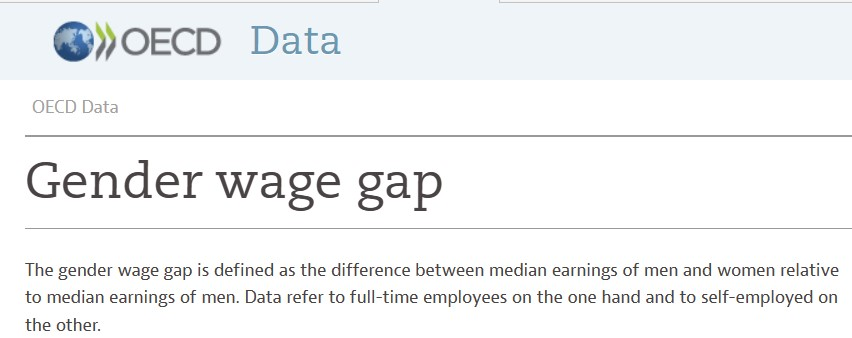Hello. In this post, I will analyze Gender wage gap of OECD data with R.

https://data.oecd.org/earnwage/gender-wage-gap.htm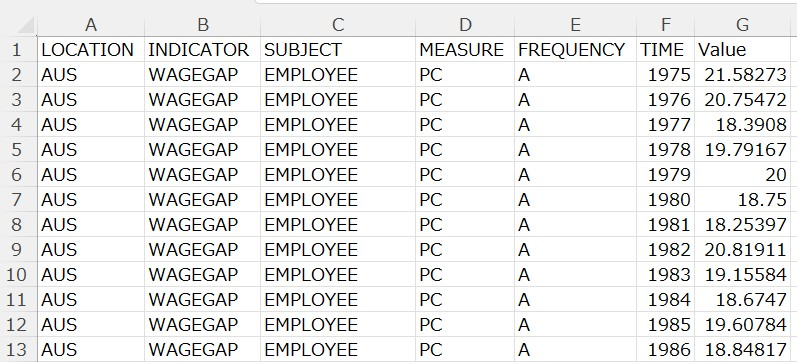I use R to analyze this data.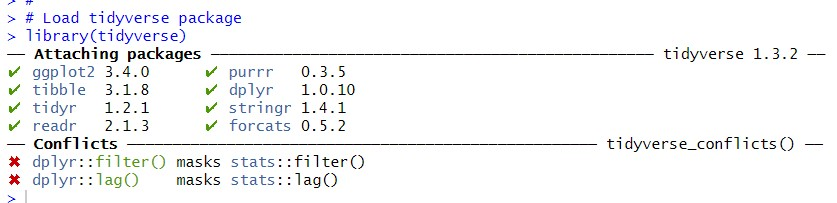Then, use read_csv() command to load the CSV file data into R.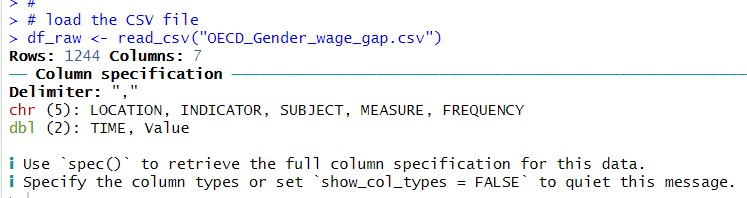I see 5 variables are categorized as chr and 2 variables are categorized as dbl.

Let's check each variables.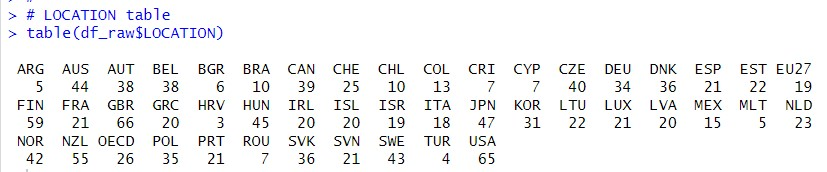LOCATION is country.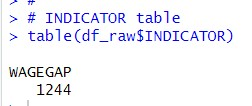INDICATOR has only one value, WAGEGAP, so I will delete this variable.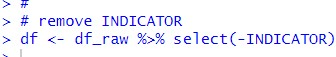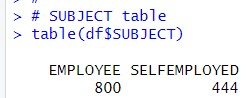SUBJECT has two value, EMPLOYEE and SELFEMPLOYED. I keep SUBJECT.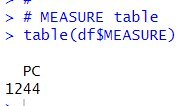MEASURE has only one value, PC. I remove MEASURE.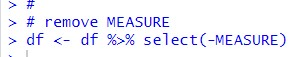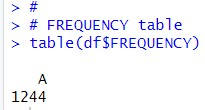FREQUENCY has only one value, A, so I remove REQUENCY.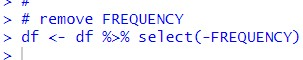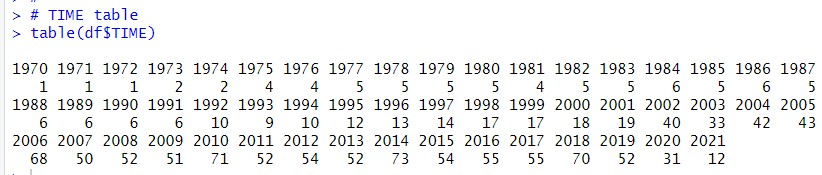The oldest TIME is 1970 and the newest TIME is 2021. 2014 has the most many observations, there are 73 observations.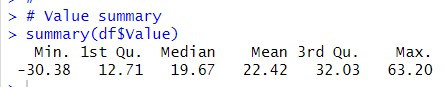Let's calculate mean Value by SUBJECT.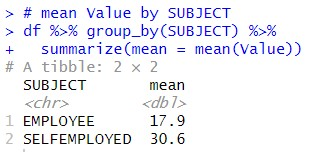I see SELFEMPLOYED mean is higher than EMPLOYEE mean. It means there is much Gender gap in SELFEMPLOYED than in EMPLOYEE.

That's it. Thank you!

Next post is